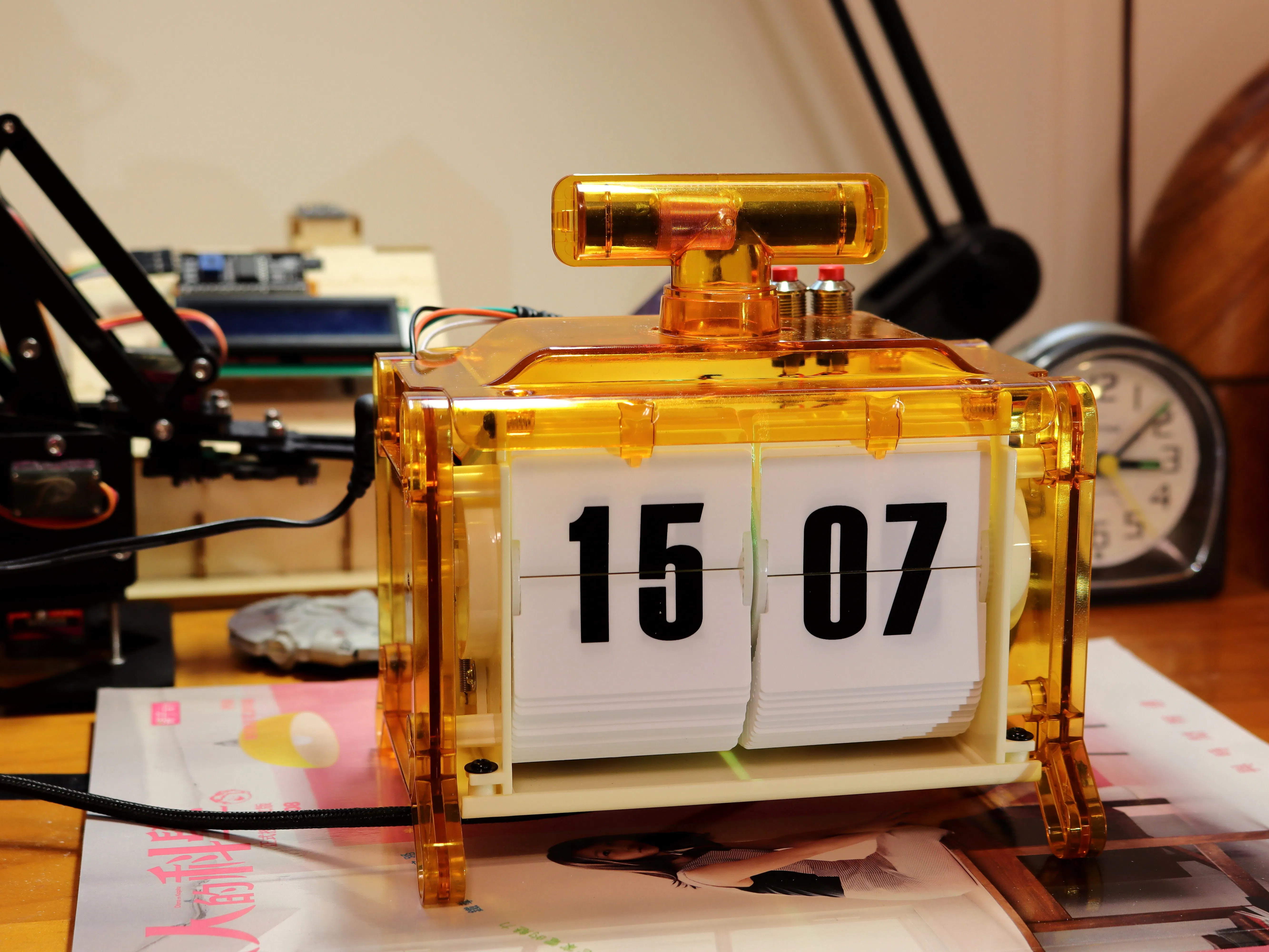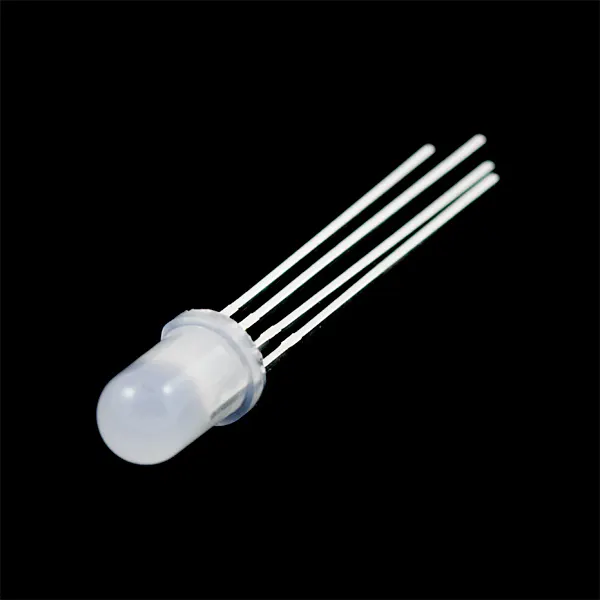# ESP32 Web Flip Clock (Modified パタパタ電波時計)

Modified the DIY split-flip display radio clock from a Japanese magazine into a true web clock.

IntermediateShowcase (no instructions)563## Things used in this project

### Hardware components

 NodeMCU-32S ESP32 board
×1
 L9110S Motor Driver Board
×1
 DS3231 RTC module
×1
 MB102 Power Supply Module
×1×1RGB Diffused Common Anode
×1

### Software apps and online servicesMicroPython

## Code

### ESP32 Web Flip Clock

MicroPython
```# ESP32 Web Flip Clock by Alan Wang

from machine import Pin, PWM, I2C, RTC
import network, urequests, utime, urandom, gc

try:
from DS3231 import DS3231
except:
print("DS3231 module import failed")

try:
from tm1637 import TM1637
except:
print("TM1637 module import failed")

utime.sleep_ms(1000)
print("System startup")

wifi = network.WLAN(network.STA_IF)
wifi.active(True)
rtc = RTC()

ssid = "your_wifi_ssid"
url = "http://worldtimeapi.org/api/timezone/Asia/Taipei"
web_query_delay = 3600000
retry_delay = 30000

hour_list = [0, 0, 1, 1, 2, 2, 3, 3, 4, 4, 5, 5, 6, 6, 7, 7, 8, 8, 9, 9, 10, 10, 11, 11, 12, 12, 12,
13, 13, 13, 14, 14, 14, 15, 15, 15, 16, 16, 16, 17, 17, 17, 18, 18, 18, 19, 19, 19,
20, 20, 20, 21, 21, 21, 22, 22, 22, 23, 23, 23]

minute_list = [0, 1, 2, 3, 4, 5, 6, 7, 8, 9, 10, 11, 12, 13, 14, 15, 16, 17, 18, 19, 20,
21, 22, 23, 24, 25, 26, 27, 28, 29, 30, 31, 32, 33, 34, 35, 36, 37, 38, 39, 40,
41, 42, 43, 44, 45, 46, 47, 48, 49, 50, 51, 52, 53, 54, 55, 56, 57, 58, 59]

hour_index = 0
minute_index = 0
time_updated = False

hour_button = Pin(32, Pin.IN, Pin.PULL_UP)
minute_button = Pin(33, Pin.IN, Pin.PULL_UP)
hour_pos_check = Pin(17, Pin.IN, Pin.PULL_UP)
minute_pos_check = Pin(16, Pin.IN, Pin.PULL_UP)

hour_motor = Pin(22, Pin.OUT)
minute_motor = Pin(21, Pin.OUT)
hour_motor.off()
minute_motor.off()

hour_pos_check_prev = hour_pos_check.value()
minute_pos_check_prev = minute_pos_check.value()
hour_pos_reached = False
minute_pos_reached = False

led_r = Pin(27, Pin.OUT)
led_g = Pin(14, Pin.OUT)
led_b = Pin(12, Pin.OUT)

def flip_hour():
hour_motor.on()
utime.sleep_ms(75)
hour_motor.off()
utime.sleep_ms(125)

def flip_minute():
minute_motor.on()
utime.sleep_ms(75)
minute_motor.off()
utime.sleep_ms(125)

led_r.off()
led_g.off()
led_b.off()
for i in range(5):
led_r.value(1 if r else 0)
led_g.value(1 if g else 0)
led_b.value(1 if b else 0)
utime.sleep_ms(50)
led_r.off()
led_g.off()
led_b.off()
utime.sleep_ms(50)
led_r.value(1 if r else 0)
led_g.value(1 if g else 0)
led_b.value(1 if b else 0)
utime.sleep_ms(500)

print("Motor calibrating...")

while True:

if hour_pos_reached and minute_pos_reached:
break

if not hour_pos_reached:
flip_hour()
if hour_pos_check_prev == 0 and hour_pos_check.value() == 1:
hour_pos_reached = True

if not minute_pos_reached:
flip_minute()
if minute_pos_check_prev == 0 and minute_pos_check.value() == 1:
minute_pos_reached = True

hour_pos_check_prev = hour_pos_check.value()
minute_pos_check_prev = minute_pos_check.value()

print("Calibrated.")

try:
tm = TM1637(clk=Pin(26), dio=Pin(25))
tm.brightness(1)
tm.numbers(0, 0)
print("TM1637 initialized")
except:
print("TM1637 initialize failed")

try:
scl_pin = Pin(19, pull=Pin.PULL_UP)
sda_pin = Pin(18, pull=Pin.PULL_UP)
i2c = I2C(-1, scl=scl_pin, sda=sda_pin)
ds3231 = DS3231(i2c)
print("DS3231 initialized")
except:
print("DS3231 initialize failed")

print("Connecting to wifi...")
wifi.connect(ssid, pw)
while not wifi.isconnected():
pass
print("Connected")

gc.enable()

def led_change():
led_r.value(urandom.getrandbits(1))
led_g.value(urandom.getrandbits(1))
if led_r.value() == 0 and led_g.value() == 0:
led_b.value(1)
else:
led_b.value(urandom.getrandbits(1))

led_change()
hour_now = 0
minute_now = 0
minute_prev = 0
update_time = utime.ticks_ms() - web_query_delay

while True:

if wifi.isconnected():

try:
tm.brightness(7)
except:
pass

if utime.ticks_ms() - update_time >= web_query_delay:

response = urequests.get(url)

if response.status_code == 200:

print("JSON query successful:")
parsed = response.json()
datetime_str = str(parsed["datetime"])
print(datetime_str)
y = int(datetime_str[0:4])
m = int(datetime_str[5:7])
d = int(datetime_str[8:10])
h = int(datetime_str[11:13])
mi = int(datetime_str[14:16])
s = int(datetime_str[17:19])
subs = int(round(int(datetime_str[20:26]) / 10000))

rtc.datetime((y, m, d, None, h, mi, s, subs))
print("RTC updated")

try:
ds3231.DateTime([y, m, d, None, h, mi, s])
print("DS3231 updated")
except:
print("DS3231 update failed")

if not time_updated:
time_updated = True

update_time = utime.ticks_ms()

else:
update_time = utime.ticks_ms() - web_query_delay + retry_delay

else:
try:
tm.brightness(1)
except:
pass

turned = False

if time_updated:

try:
hour_now = ds3231.DateTime()
minute_now = ds3231.DateTime()
rtc.datetime((ds3231.DateTime(),
ds3231.DateTime(),
ds3231.DateTime(),
ds3231.DateTime(),
ds3231.DateTime(),
ds3231.DateTime(),
ds3231.DateTime(),
0
))
except:
hour_now = rtc.datetime()
minute_now = rtc.datetime()

try:
tm.numbers(hour_now, minute_now)
except:
pass

if hour_list[hour_index] != hour_now:
led_change()
flip_hour()
hour_index += 1
if hour_index >= 60:
hour_index = 0
turned = True

if minute_list[minute_index] != minute_now:
led_change()
flip_minute()
minute_index += 1
if minute_index >= 60:
minute_index = 0
turned = True

if hour_button.value() == 0:
led_change()
flip_hour()
utime.sleep_ms(250)
turned = True

if minute_button.value() == 0:
led_change()
flip_minute()
utime.sleep_ms(250)
turned = True

if not turned:
utime.sleep_ms(250)
```

## Credits

### Alan Wang

25 projects • 42 followers
Former novel translator, amateur film photographer. Sometime Maker.